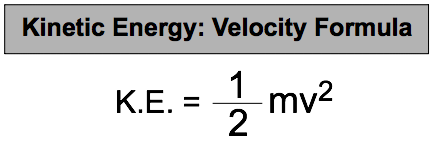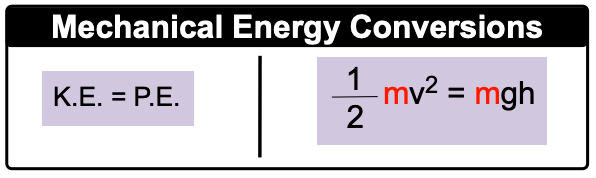Clutch Prep is now a part of Pearson
Ch.6 - Thermochemistry WorksheetSee all chapters

# Kinetic & Potential Energy

See all sections
Sections
Nature of Energy
Kinetic & Potential Energy
First Law of Thermodynamics
Internal Energy
Endothermic & Exothermic Reactions
Heat Capacity
Constant-Pressure Calorimetry
Constant-Volume Calorimetry
Thermal Equilibrium
Thermochemical Equations
Formation Equations
Enthalpy of Formation
Hess's Law

Mechanical Energy is the energy associated with an object's velocity (Kinetic Energy) and its position (Potential Energy).

###### Kinetic Energy & Potential Energy

Concept #1: Kinetic & Potential Energy

The kinetic energy of a gas molecule is connected to its mass in kilograms and velocity in meters per second.The potential energy of a gas molecule is connected to its mass, acceleration due to gravity and height above the ground.Example #1: Calculate the kinetic energy (in kJ) of an electron (m = 9.11 x 10-31 kg) moving at 1.59 x 1020 m/s.

Practice: A radioactive particle weighing 7.20 x 103 ng is found 110 m above the earth’s surface. What is its potential energy?

Concept #2: Energy InterconversionsExample #2: A neutron weighing 1.67 x 10-27 kg is shot from a laser projector that is mounted 120.0 meters above the ground. What is its speed when it hits the ground?